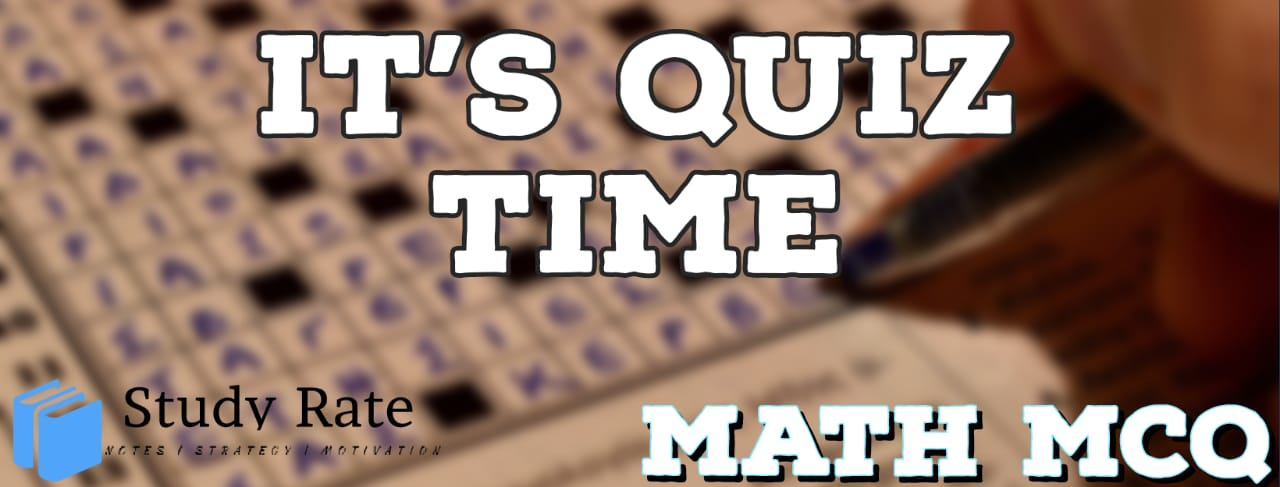## CBSE Class 10 Maths Chapter 9 Application of Trigonometry MCQ Quiz

Free PDF Download of CBSE Class 10 Maths Chapter 9 Application of Trigonometry Multiple Choice Questions with Answers. MCQ Questions for Class 10 Maths with Answers was Prepared Based on Latest Exam Pattern. Students can solve Class 10 Maths Application of Trigonometry MCQs with Answers to know their preparation level

Applications of Trigonometry CBSE Class 10 Math’s MCQ Quiz

1. A Technician has to repair a light on a pole of height 10 m. She needs to reach a point 1 m
below the top of the pole to undertake the repair work. What should be the length of the
ladder that she should use which, when inclined at an angle of 60∘ to the ground, would
enable her to reach the required position? Also, how far from the foot of the pole should
she place the foot of the ladder?

2. If the length of the shadow of a tower is increasing, then the angle of elevation of the sun

3. The angle of elevation of the top of a tower is 30°. If the height of the tower is doubled, then the angle of elevation of its top will

4. If the height of a tower and the distance of the point of observation from its foot, both, are increased by 10%, then the angle of elevation of its top

5. A ladder 15 metres long just reaches the top of a vertical wall. If the ladder makes an angle of 60° with the wall, then the height of the wall will be

6. A circus artist is climbing a 20 m long rope, which is tightly stretched and tied from the top of a vertical pole to the ground. Find the height of the pole if the angle made by the rope with the ground level is 30°

7. A contractor plans to install two slides for the children to play in a park. For the children below the age of 5 years, she prefers to have a slide whose top is at a height of 2m and is inclined at an angle of 30° to the ground. What should be the length of the slide?

8. If a man standing on a platform 3 metres above the surface of a lake observes a cloud and its reflection in the lake, then the angle of elevation of the cloud is

9. The angle of elevation of the top of a tower from certain point is 30°. If the observer moves 20 metres towards the tower, the angle of elevation of the top increases by 15°. Find the height of the tower

10. A vertical tower stands on a horizontal plane and is surmounted by a vertical flag staff of height h. At a point on the plane, the angles of elevation of the bottom and the top of the flag staff are α and β, respectively. Then the height of the tower is: